# 线性回归(Linear Regression with One Variable)

wuchangjian2021-10-30 21:02:47编程学习

## 2.1 线性回归算法模型表示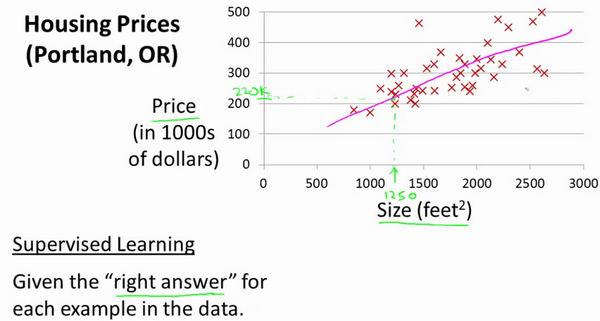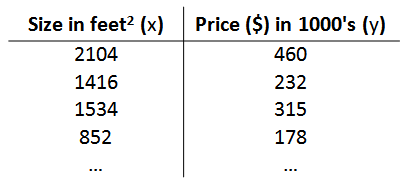m 代表训练集中实例的数量

x 代表特征/输入变量

y 代表目标变量/输出变量

(x,y)代表训练集中的实例

(x(i),y(i))代表第i个观察实例

h代表学习算法的解决方案或函数也称为假设（hypothesis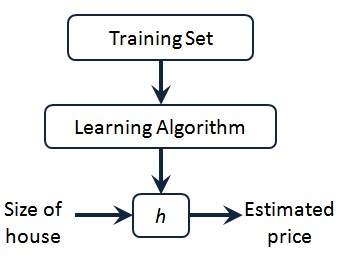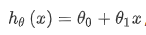### 2.2 代价函数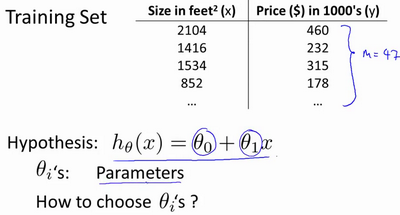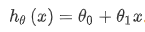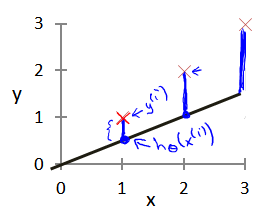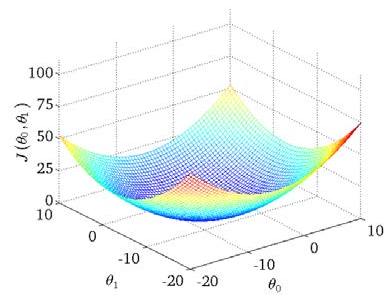# 通过穷举法实现实现寻找最低点or最小误差

代码：

``````import numpy as np
import matplotlib.pyplot as plt

#数据集
x_data=[1.0,2.0,3.0]
y_data=[2.0,4.0,6.0]

#构建 y = w * x ===>预测值
def forward(x):
return x*w

#计算loss 即 loss 公式如上图，预测值减去原始数据求误差
def loss(x,y):
y_pred=forward(x)
return (y_pred-y)*(y_pred-y)
#权重w的列表
w_list=[]
#损失loss的列表
mse_list=[]

#对w使用穷举法，计算机y=w*x的最佳拟合值
for w in np.arange(0.0,4.1,0.1):
print(w)
l_sum=0
#zip()当传递参数长度不一样时候，以短的为标准
for x_val,y_val in zip(x_data,y_data):
#计算新的预测值
y_pred_val=forward(x_val)
#新预测值与
loss_val=loss(x_val,y_val)
l_sum= l_sum+loss_val
print(x_val,y_val,y_pred_val,loss_val)
#除以样本数，即平均误差
MSR=l_sum/3
w_list.append(w)
mse_list.append(MSR)
#绘制损失loss与权重w之间的函数图像
plt.plot(w_list,mse_list)
plt.ylabel('Loss')
plt.xlabel('w')
plt.show()
``````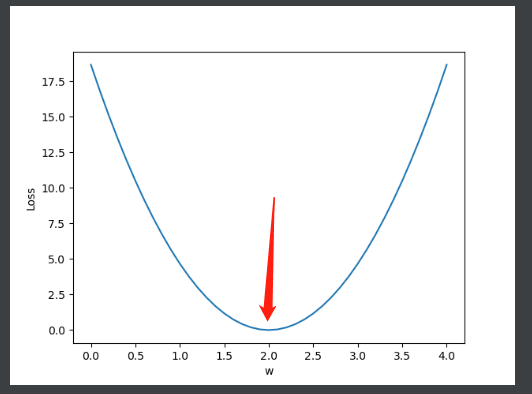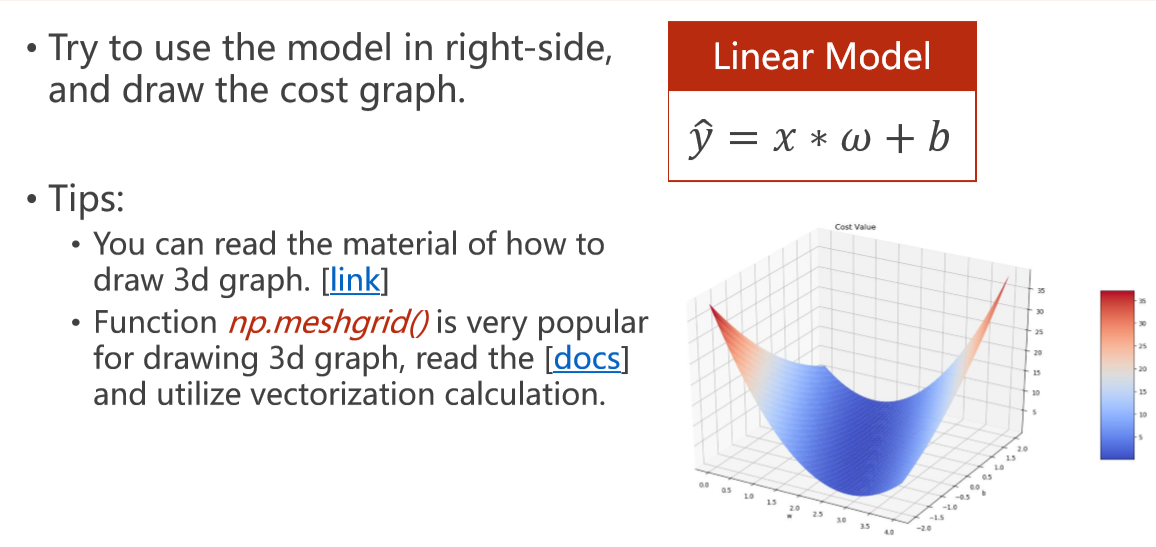``````import numpy as np
import matplotlib.pyplot as plt
from mpl_toolkits.mplot3d import Axes3D

#数据集
x_data = [1.0,2.0,3.0]
y_data = [5.0,8.0,11.0]

# 数据集长度
m=len(x_data)

# 权值
W=np.arange(0.0,4.0,0.1)
B=np.arange(-2.0,2.0,0.1)
[w,b]=np.meshgrid(W,B)
#即三维坐标下 W做X周 B做Y周，在绘图时候一定要用小写，因为小写是N×N的二维数组，大写是一维N个数组成的数组

# 注意矩阵的运算
def farword(x):
return x * w+b

def loss(y_test,y):
return (y_test-y)*(y_test-y)

total_loss = 0
for x_val,y_val in zip(x_data,y_data):
y_test=farword(x_val)
total_loss=(total_loss+loss(y_test,y_val))/m

fig = plt.figure()

# MatplotlibDeprecationWarning: Calling gca() with keyword arguments was deprecated in Matplotlib 3.4. Starting two minor releases later, gca() will take no keyword arguments. The gca() function should only be used to get the current axes, or if no axes exist, create new axes with default keyword arguments. To create a new axes with non-default arguments, use plt.axes() or plt.subplot().
# ax = fig.gca(projection='3d') 改为
ax = Axes3D(fig)

print(W.shape) #(40,)
print(W)
print(w.shape)#(40,40)
print(w)

# ax.plot_surface(W,B,total_loss)
ax.plot_surface(w,b,total_loss)
plt.show()``````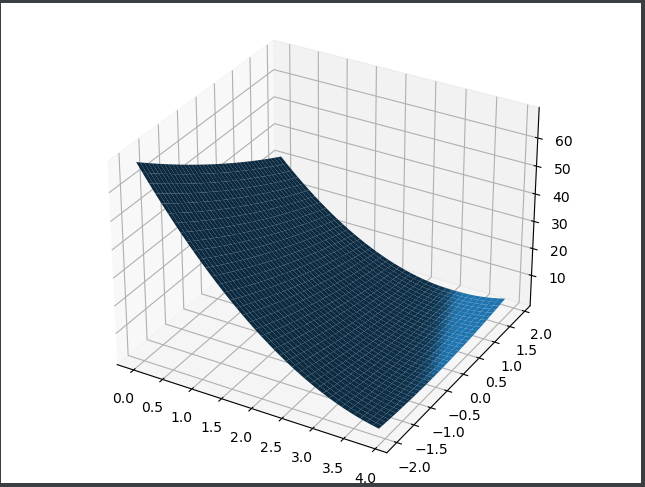numpy.meshgrid()理解

numpy.meshgrid()理解_lllxxq141592654的博客-CSDN博客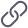https://blog.csdn.net/lllxxq141592654/article/details/81532855

...

...

### 设计模式——（12）代理模式

1、定义 由于某些原因需要给某对象提供一个代理以控制对该对象的访问。这时，...

### 二维列表python

h_phone=['p','mate','...

#### 发表评论◎欢迎参与讨论，请在这里发表您的看法、交流您的观点。